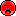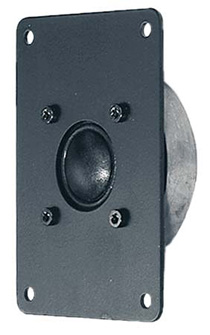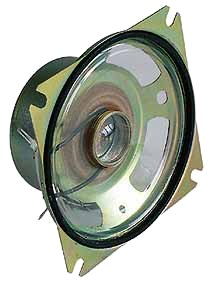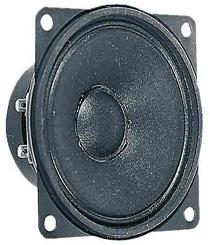# Visaton

With 40 years of experience in the field of acoustics and loudspeaker technology Visaton is a future oriented Company. With high engagement in research and development plus the high quality standards of production, Visaton is a specialised and world wide successfully operating German loudspeaker manufacturer.

## no longer sold## Visaton UL7N 100V

order no. V-50471- not available

cm full range driver with high quality cone.

• power handling (continuous/programme) = 10/5/2,5/1,5/0,8 W
• frequency range = 300-20000 Hz
• impedance R = 1,0; 2,0; 4,0; 6,6; 12,5 kOhm Ohm
• sound pressure level SPL = 90 dB (2,83V; 1m)
• overall diameter d = 72x280 mm
• mounting depth (not countersunk) t = 135,5 mm
• mass m = 1,45 kg

## Visaton UL7NMB

order no. V-50476- not available

.

• overall diameter d = 40x191 mm
• mounting depth (not countersunk) t = 59 mm
• mass m = 0,214 kg

## Visaton PL5 8 Ohm

order no. V-4466- not available

.

• power handling (continuous/programme) = `4/10 W
• frequency range = 350-17000 Hz
• resonance frequency fs = 550 Hz
• impedance R = 8 Ohm
• sound pressure level SPL = 83 dB (2,83V; 1m)
• overall diameter d = 77 mm
• mounting depth (not countersunk) t = 50 mm
• mass m = 0,194 kg

## Visaton WL13PRP 100V

order no. V-50324- not available

28x17 cm full range driver with high quality cone.

• power handling (continuous/programme) = 6/3/1,5 W
• frequency range = 90-16000 Hz
• sound pressure level SPL = 88 dB (2,83V; 1m)
• overall diameter d = 170x280 mm
• mounting depth (not countersunk) t = 61 mm

## Visaton WL13NRP 100V

order no. V-50304- not available

.

• power handling (continuous/programme) = 6/3/1,5 W
• frequency range = 90-20000 Hz
• sound pressure level SPL = 88 dB (2,83V; 1m)
• overall diameter d = 170x280 mm
• mounting depth (not countersunk) t = 65 mm
• mass m = 1,4 kg

## Visaton WL13NAB 100V

order no. V-50306- not available

28x17 cm full range driver with high quality cone.

• power handling (continuous/programme) = 2x3/2x1,8/2x0,75 W
• frequency range = 120-16000 Hz
• sound pressure level SPL = 88 dB (2,83V; 1m)
• overall diameter d = 170x280 mm
• mounting depth (not countersunk) t = 65 mm
• mass m = 1,68 kg

## Visaton ML16 100V

order no. V-50131- not available

. The diaphragm is varnished with a hydrophabic coat.

• power handling (continuous/programme) = 2/1/0,5 W
• frequency range = 100-15000 Hz
• resonance frequency fs = 150 Hz
• impedance R = 5/10/20 (kohm) Ohm
• sound pressure level SPL = 94 dB (2,83V; 1m)
• voice coil diameter = 14 mm
• mounting diameter d = 143 mm
• overall diameter d = 170 mm
• mounting depth (not countersunk) t = 65 mm
• mass m = 1,1 kg

## Visaton DX4x6P 4 Ohm

order no. V-4660- not available

15x9 cm coax driver with polypropylene cone.

• power handling (continuous/programme) = 30/50 W
• frequency range = 65-22000 Hz
• resonance frequency fs = 90 Hz
• impedance R = 4 Ohm
• sound pressure level SPL = 89 dB (2,83V; 1m)
• voice coil diameter = `20/14 mm
• maximum peak linear excursion vibration xlin = +/- 1,5 mm
• mounting diameter d = 88x144 mm
• overall diameter d = 97,5x153 mm
• mounting depth (not countersunk) t = 48 mm

## Visaton FR10WPX 4 Ohm weiß

order no. V-2150- not available

11 cm full range driver with high quality cone.

• power handling (continuous/programme) = 20/30 W
• frequency range = 80-16000 Hz
• resonance frequency fs = 120 Hz
• impedance R = 4 Ohm
• sound pressure level SPL = 83 dB (2,83V; 1m)
• voice coil diameter = 20 mm
• mounting diameter d = 101 mm
• overall diameter d = 132 mm
• mounting depth (not countersunk) t = 59 mm
• mass m = 0,275 kg

## Visaton FR10WPX 4 Ohm schwarz

order no. V-2180- not available

11 cm full range driver with high quality cone.

• power handling (continuous/programme) = 20/30 W
• frequency range = 80-16000 Hz
• resonance frequency fs = 120 Hz
• impedance R = 4 Ohm
• sound pressure level SPL = 83 dB (2,83V; 1m)
• voice coil diameter = 20 mm
• mounting diameter d = 101 mm
• overall diameter d = 132 mm
• mounting depth (not countersunk) t = 59 mm
• mass m = 0,275 kgrecommended cabinet 1:
closed cabinet with 36 L volume
from 101/64 Hz (-3dB/-8dB)

recommended cabinet 2:
106 L volume bass reflex cabinet
with 2x HP100 reflex tube, 6 cm long.
from 56/43 Hz (-3dB/-8dB).

## Visaton BGS 40 8 Ohm

order no. V-3040- not available

38 cm bass driver with high quality cone.

• power handling (continuous/programme) = 300/700 W
• frequency range = 40-4000 Hz
• resonance frequency fs = 38 Hz
• impedance R = 8 Ohm
• sound pressure level SPL = 98 dB (2,83V; 1m)
• DC resistance Re = 6,5 Ohm
• force factor BL = 16,8 N/A
• voice coil inductance L = 1,55 mH
• effective piston radiating area Sd = 855 cm2
• effective mechanical mass incl. air load mms = 80 g
• equivalent volume of compliance Vas = 263 l
• total Q factor Qts = 0,31 (Qms=1,3, Qes=0,4)
• voice coil diameter = 65 mm
• maximum peak linear excursion vibration xlin = +/- 2 mm
• mounting diameter d = 359 mm
• overall diameter d = 403 mm
• mounting depth (not countersunk) t = 147 mmopen a bigger photo

recommended cabinet 1:
closed cabinet with 30 L volume
from 70/44 Hz (-3dB/-8dB)

recommended cabinet 2:
77 L volume bass reflex cabinet
with 2x HP100 reflex tube, 36 cm long.
from 41/31 Hz (-3dB/-8dB).

## Visaton W300S 4 Ohm

order no. V-9040- not available

31 cm bass driver with high quality cone.

• power handling (continuous/programme) = 150/200 W
• frequency range = -4200 Hz
• resonance frequency fs = 23 Hz
• impedance R = 4 Ohm
• sound pressure level SPL = 92 dB (2,83V; 1m)
• DC resistance Re = 3,5 Ohm
• force factor BL = 8,4 N/A
• voice coil inductance L = 1,1 mH
• effective piston radiating area Sd = 507 cm2
• effective mechanical mass incl. air load mms = 62 g
• equivalent volume of compliance Vas = 300 l
• total Q factor Qts = 0,25 (Qms=1,6, Qes=0,3)
• voice coil diameter = 50 mm
• maximum peak linear excursion vibration xlin = +/- 14 mm
• mounting diameter d = 280 mm
• overall diameter d = 332 mm
• mounting depth (not countersunk) t = 144 mm
• mass m = 4,5 kgopen a bigger photo

recommended cabinet 1:
closed cabinet with 58 L volume
from 56/35 Hz (-3dB/-8dB)

recommended cabinet 2:
187 L volume bass reflex cabinet
with 2x HP100 reflex tube, 19 cm long.
from 30/23 Hz (-3dB/-8dB).

## Visaton W300S 8 Ohm

order no. V-9041- not available

31 cm bass driver with high quality cone.

• power handling (continuous/programme) = 150/200 W
• frequency range = -4200 Hz
• resonance frequency fs = 23 Hz
• impedance R = 8 Ohm
• sound pressure level SPL = 92 dB (2,83V; 1m)
• DC resistance Re = 6,7 Ohm
• force factor BL = 11 N/A
• voice coil inductance L = 1,8 mH
• effective piston radiating area Sd = 507 cm2
• effective mechanical mass incl. air load mms = 57 g
• equivalent volume of compliance Vas = 340 l
• total Q factor Qts = 0,33 (Qms=1,65, Qes=0,42)
• voice coil diameter = 50 mm
• maximum peak linear excursion vibration xlin = +/- 14 mm
• mounting diameter d = 280 mm
• overall diameter d = 332 mm
• mounting depth (not countersunk) t = 144 mm
• mass m = 4,5 kg

## Visaton DSM25R 8 Ohm

order no. V-1155- not available

25 mm dome tweeter with titanium dome. Voice coil windings immersed in magnetic fluid increase short term power handling capacity.

• power handling (continuous/programme) = 80/140 W
• frequency range = 1000-30000 Hz
• resonance frequency fs = 1600 Hz
• impedance R = 8 Ohm
• sound pressure level SPL = 90 dB (2,83V; 1m)
• DC resistance Re = 5,9 Ohm
• voice coil inductance L = 5,9 mH
• effective piston radiating area Sd = 4,9 cm2
• equivalent volume of compliance Vas = 0,92 l
• total Q factor Qts = 0,92 (Qms=1,83, Qes=1,87)
• voice coil diameter = 25 mm
• mounting diameter d = 80 mm
• overall diameter d = 104,5 mm
• mounting depth (not countersunk) t = 31 mm
• mass m = 0,53 kg## Visaton SC4ND

order no. V-8054- not available

25 mm tweeter with high quality cone.

• power handling (continuous/programme) = 80/120 W
• frequency range = 1000-22000 Hz
• resonance frequency fs = 1300 Hz
• impedance R = 4 Ohm
• sound pressure level SPL = 90 dB (2,83V; 1m)
• voice coil diameter = 25 mm
• mounting diameter d = 50 mm
• overall diameter d = 50 mm
• mounting depth (not countersunk) t = 15 mm## Visaton DTW8.12NG

order no. V-1033

- not available (_)

25 mm tweeter with high quality cone.

• power handling (continuous/programme) = 110/150 W
• frequency range = 1000-22000 Hz
• resonance frequency fs = 1500 Hz
• impedance R = 8 Ohm
• sound pressure level SPL = 90 dB (2,83V; 1m)
• voice coil diameter = 25 mm
• mounting diameter d = 80 mm
• overall diameter d = 115x82 mm
• mounting depth (not countersunk) t = 24 mm## Visaton G25ND

order no. V-1199

- not available (_)

25 mm tweeter with high quality cone.

• power handling (continuous/programme) = 80/120 W
• frequency range = 1000-30000 Hz
• resonance frequency fs = 1500 Hz
• impedance R = 8 Ohm
• sound pressure level SPL = 89 dB (2,83V; 1m)
• voice coil diameter = 25 mm
• mounting diameter d = 45 mm
• overall diameter d = 104x52 mm
• mounting depth (not countersunk) t = 10 mm## Visaton F8SC

order no. V-8027

- not available (_)

8 cm full range driver with high quality cone.

• power handling (continuous/programme) = 20/30 W
• frequency range = 80-15000 Hz
• resonance frequency fs = 103 Hz
• impedance R = 4 Ohm
• sound pressure level SPL = 82 dB (2,83V; 1m)
• DC resistance Re = 3,4 Ohm
• force factor BL = 2,1 N/A
• voice coil inductance L = 0,18 mH
• effective piston radiating area Sd = 30 cm2
• effective mechanical mass incl. air load mms = 2,2 g
• equivalent volume of compliance Vas = 1,4 l
• total Q factor Qts = 1,01 (Qms=3,03, Qes=1,52)
• voice coil diameter = 20 mm
• maximum peak linear excursion vibration xlin = +/- 0,8 mm
• mounting diameter d = 78 mm
• overall diameter d = 94 mm
• mounting depth (not countersunk) t = 42 mm## Visaton SL87WP

order no. V-2067

- not available (_)

9 cm full range driver with high quality cone.

• power handling (continuous/programme) = 20/30 W
• frequency range = 180-10000 Hz
• resonance frequency fs = 270 Hz
• impedance R = 4 Ohm
• sound pressure level SPL = 87 dB (2,83V; 1m)
• DC resistance Re = 3,8 Ohm
• effective piston radiating area Sd = 41 cm2
• total Q factor Qts = 2,16 (Qms=6,91, Qes=3,14)
• voice coil diameter = 20 mm
• mounting diameter d = 80 mm
• overall diameter d = 100 mm
• mounting depth (not countersunk) t = 38 mm## Visaton SL87WP-8

order no. V-2068

- not available (_)

9 cm full range driver with high quality cone.

• power handling (continuous/programme) = 20/30 W
• frequency range = 180-10000 Hz
• resonance frequency fs = 270 Hz
• impedance R = 8 Ohm
• sound pressure level SPL = 87 dB (2,83V; 1m)
• DC resistance Re = 3,8 Ohm
• effective piston radiating area Sd = 41 cm2
• total Q factor Qts = 2,16 (Qms=6,91, Qes=3,14)
• voice coil diameter = 20 mm
• mounting diameter d = 80 mm
• overall diameter d = 100 mm
• mounting depth (not countersunk) t = 38 mm## Visaton M10-4

order no. V-9189- not available

10 cm midrange driver with high quality cone.

• power handling (continuous/programme) = 80/100 W
• frequency range = 450-13000 Hz
• resonance frequency fs = 700 Hz
• impedance R = 4 Ohm
• sound pressure level SPL = 90 dB (2,83V; 1m)
• voice coil diameter = 20 mm
• mounting diameter d = 95 mm
• overall diameter d = 117 mm
• mounting depth (not countersunk) t = 39 mmrecommended cabinet 1:
closed cabinet with 4,3 L volume
from 117/74 Hz (-3dB/-8dB)

recommended cabinet 2:
17 L volume bass reflex cabinet
with HP50 reflex tube, 5 cm long.
from 52/46 Hz (-3dB/-8dB).

## Visaton TI100M

order no. V-1273- not available

11 cm midrange driver with high quality cone.

• power handling (continuous/programme) = 30/50 W
• frequency range = 70-20000 Hz
• resonance frequency fs = 84 Hz
• impedance R = 8 Ohm
• sound pressure level SPL = 89 dB (2,83V; 1m)
• DC resistance Re = 6 Ohm
• force factor BL = 4 N/A
• voice coil inductance L = 0,6 mH
• effective piston radiating area Sd = 54 cm2
• effective mechanical mass incl. air load mms = 3 g
• equivalent volume of compliance Vas = 4,9 l
• total Q factor Qts = 0,56 (Qms=6,33, Qes=0,61)
• voice coil diameter = 20 mm
• maximum peak linear excursion vibration xlin = +/- 1 mm
• mounting diameter d = 100 mm
• overall diameter d = 122 mm
• mounting depth (not countersunk) t = 72 mmrecommended cabinet 1:
closed cabinet with 9,4 L volume
from 77/48 Hz (-3dB/-8dB)

recommended cabinet 2:
37 L volume bass reflex cabinet
with HP50 reflex tube, 6 cm long.
from 34/30 Hz (-3dB/-8dB).

## Visaton W130SE

order no. V-9053- not available

12 cm midrange driver with paper cone.

• power handling (continuous/programme) = 50/80 W
• frequency range = 55-12000 Hz
• resonance frequency fs = 54 Hz
• impedance R = 8 Ohm
• sound pressure level SPL = 87 dB (2,83V; 1m)
• DC resistance Re = 6,2 Ohm
• force factor BL = 4,2 N/A
• effective piston radiating area Sd = 74 cm2
• effective mechanical mass incl. air load mms = 6 g
• equivalent volume of compliance Vas = 11,5 l
• total Q factor Qts = 0,56 (Qms=2,68, Qes=0,71)
• voice coil diameter = 25 mm
• mounting diameter d = 106 mm
• overall diameter d = 131 mm
• mounting depth (not countersunk) t = 55 mmrecommended cabinet 1:
closed cabinet with 1,7 L volume
from 125/79 Hz (-3dB/-8dB)

recommended cabinet 2:
6,8 L volume bass reflex cabinet
with HP50 reflex tube, 16 cm long.
from 61/50 Hz (-3dB/-8dB).

## Visaton W100SC

order no. V-9120- not available

11 cm bass driver with high quality cone.

• power handling (continuous/programme) = 30/50 W
• frequency range = 70-15000 Hz
• resonance frequency fs = 67 Hz
• impedance R = 4 Ohm
• sound pressure level SPL = 86 dB (2,83V; 1m)
• DC resistance Re = 3,5 Ohm
• force factor BL = 3,4 N/A
• voice coil inductance L = 0,47 mH
• effective piston radiating area Sd = 53 cm2
• effective mechanical mass incl. air load mms = 5,4 g
• equivalent volume of compliance Vas = 5,2 l
• total Q factor Qts = 0,43 (Qms=1,97, Qes=0,55)
• voice coil diameter = 20 mm
• maximum peak linear excursion vibration xlin = +/- 2 mm
• mounting diameter d = 94 mm
• overall diameter d = 122 mm
• mounting depth (not countersunk) t = 61 mmrecommended cabinet 1:
closed cabinet with 1,8 L volume
from 123/77 Hz (-3dB/-8dB)

recommended cabinet 2:
7,3 L volume bass reflex cabinet
with HP50 reflex tube, 15 cm long.
from 59/48 Hz (-3dB/-8dB).

## Visaton W100SC-8

order no. V-9121- not available

11 cm bass driver with high quality cone.

• power handling (continuous/programme) = 30/50 W
• frequency range = 70-15000 Hz
• resonance frequency fs = 67 Hz
• impedance R = 8 Ohm
• sound pressure level SPL = 86 dB (2,83V; 1m)
• DC resistance Re = 3,5 Ohm
• force factor BL = 3,4 N/A
• voice coil inductance L = 0,47 mH
• effective piston radiating area Sd = 53 cm2
• effective mechanical mass incl. air load mms = 3,8 g
• equivalent volume of compliance Vas = 5,2 l
• total Q factor Qts = 0,43 (Qms=1,97, Qes=0,55)
• voice coil diameter = 20 mm
• maximum peak linear excursion vibration xlin = +/- 2 mm
• mounting diameter d = 94 mm
• overall diameter d = 122 mm
• mounting depth (not countersunk) t = 61 mmrecommended cabinet 1:
closed cabinet with 3,9 L volume
from 104/65 Hz (-3dB/-8dB)

recommended cabinet 2:
13,9 L volume bass reflex cabinet
with HP50 reflex tube, 11 cm long.
from 52/42 Hz (-3dB/-8dB).

## Visaton W130SC

order no. V-9122- not available

13 cm bass driver with high quality cone.

• power handling (continuous/programme) = 50/80 W
• frequency range = 55-12000 Hz
• resonance frequency fs = 49 Hz
• impedance R = 4 Ohm
• sound pressure level SPL = 87 dB (2,83V; 1m)
• DC resistance Re = 3,7 Ohm
• force factor BL = 3,6 N/A
• voice coil inductance L = 0,55 mH
• effective piston radiating area Sd = 79 cm2
• effective mechanical mass incl. air load mms = 7,3 g
• equivalent volume of compliance Vas = 16 l
• total Q factor Qts = 0,37 (Qms=2,58, Qes=0,43)
• voice coil diameter = 25 mm
• maximum peak linear excursion vibration xlin = +/- 2,3 mm
• mounting diameter d = 108 mm
• overall diameter d = 146 mm
• mounting depth (not countersunk) t = 67 mmrecommended cabinet 1:
closed cabinet with 3,6 L volume
from 106/67 Hz (-3dB/-8dB)

recommended cabinet 2:
12,8 L volume bass reflex cabinet
with HP50 reflex tube, 11 cm long.
from 54/43 Hz (-3dB/-8dB).

## Visaton W130SC-8

order no. V-9123- not available

13 cm bass driver with high quality cone.

• power handling (continuous/programme) = 50/80 W
• frequency range = 55-12000 Hz
• resonance frequency fs = 49 Hz
• impedance R = 8 Ohm
• sound pressure level SPL = 87 dB (2,83V; 1m)
• DC resistance Re = 3,7 Ohm
• force factor BL = 3,6 N/A
• voice coil inductance L = 0,55 mH
• effective piston radiating area Sd = 79 cm2
• effective mechanical mass incl. air load mms = 5,5 g
• equivalent volume of compliance Vas = 16 l
• total Q factor Qts = 0,37 (Qms=2,58, Qes=0,43)
• voice coil diameter = 25 mm
• maximum peak linear excursion vibration xlin = +/- 2,3 mm
• mounting diameter d = 108 mm
• overall diameter d = 146 mm
• mounting depth (not countersunk) t = 67 mm

The cabinet volume calculations are executed considering an external resistance of 0.3 Ohm. We are using, if no other data is known or available, the information supplied by the manufacturer.

Please note: the frequency response doesn't say much about the cut-off frequency inside the cabinet! Therefore, we mostly give you 2 examples with dimensioning considerations and an indication, how low the loudspeaker will reproduce.

Home